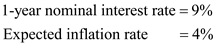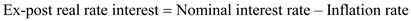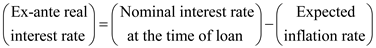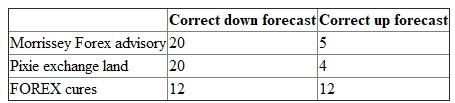## Quiz 10 : Exchange Rate Determination and ForecastingLooking for Finance Homework Help?# Quiz 10 : Exchange Rate Determination and Forecasting

Nominal interest rate and the inflation rate in Zooropa are being provided in order to determine the real interest rate.Expected real interest rate can be determined by subtracting the inflation from the nominal interest rate.Thus, the real interest rate is 5%. Alternatively the expected real interest rate can be computed using the below formula based on Fishers method.Thus, the real interest rate is 4.81%.

A lender always has more concern about the real return on investment. It is the return which actually measures the increase in the purchasing power between the two periods. The real return is determined by dividing the real amount realized by the investment by the real amount of investment made. In short the ex post real rate of return is expressed as shown below.Generally, the inflation rate is uncertain at the time when the investment is made, which makes the lenders to be unaware about the real rate of return realized from the loan. The ex ante real interest is determined using the following equation.Thus, the main difference between them is that, the ex-post interest rate actually corrects nominal interest rate with the ex post or the realized inflation rate. On the other hand, the ex-ante real interest rate will correct the nominal rate of interest for the expected inflation rate.

Analysis about overseas expansion in Europe has to be made, for which details are being provided for determining the analysing ability of the company and required additional information. Following are synopsis of given information:a. From the given information it is clear that one can determine number of correct directional forecast. Morrissey Forex advisory holds highest proportion of correct that is 25 out of 50 when compared to the other two firms and they are less than 50%. From the observation it is clear that US dollar was quite strong and appreciation, it was dominated over the depreciation against euro. Thus, any forecast with regard to down period will add more value. If correct conditional forecasts is being focused the percentage of correct forecast for Morrissey Forex advisory was about 57.14% (i.e. 20/35) when dollar appreciates but only 33.33% (i.e. 5/15) in case of dollar depreciation. If Henriksson - Merton test is considered then the sum of two proportions must be above 1 for the firm to have the ability of market timing. The total percentage is 90.47% (i.e. 57.14% + 33.33%). From this it is clear that it is better than Pixie exchange land but unable to determine with regard to FOREX cures. FOREX cures percentage has to be determined in case of appreciation it is 34.28% (i.e. 12/35) and 80% (i.e. 12/15) in case of depreciation which totals 114.28% (i.e. 34.28% + 80%). Thus, FOREX cures only disclose the directional forecasting ability. b. In case of Forex market, directional forecasting ability is not more useful if in case the forecast are being used for the market speculation. It will be more useful to know about the firms that were making forecasting were actually on the correct side with regard to forward rate. Full record related to forecast is actually required for better analysis. With the complete details Root Mean Squared Error (RMSE) or the Mean Absolute Deviation and risk-return statistic that is Sharpe ratio can be calculated.

There is no answer for this question